## Decimals: Tenths, Hundredths and Thousandths

#### 1. Understanding Tenths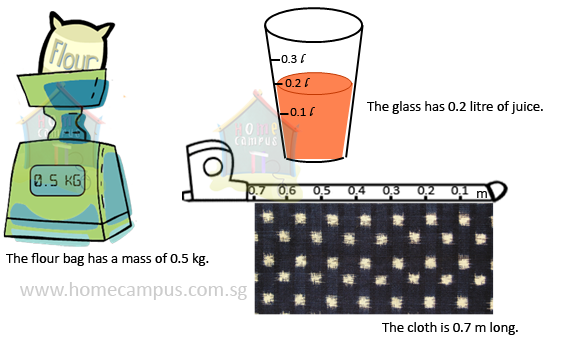0.5, 0.7 and 0.2 are decimals or decimal numbers.

Divide 1 whole into 10 equal parts.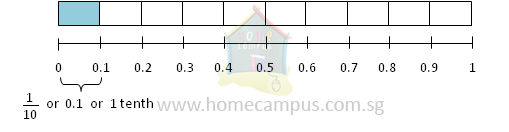In the fraction form, each part has a value equal to
 1 10
or 1 tenth.
In the decimal form, each part has a value equal to 0.1 (read as zero point one).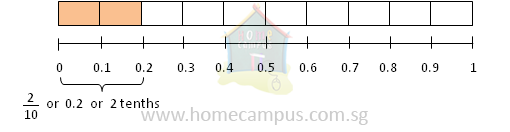Two parts are
 2 10
or 2 tenths or 0.2.
The dot in a decimal number is called a decimal point.

#### 2. What decimals do the following shaded parts represent?

a)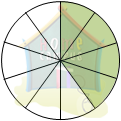4 tenths
 4 10
= 0.4
b)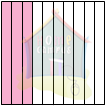3 tenths
 3 10
=  0.3
c)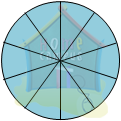10 tenths
10 tenths  =  1 whole
=  1
10 tenths make 1 whole.
d)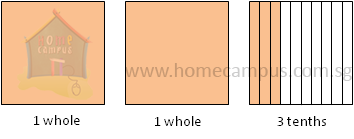2 3 10
=   2  +
 3 10

=  2  +  0.3
=  2.3
e)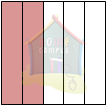2 fifths
 2 5
=
 4 10

=    0.4
2 fifths  =  4 tenths

a)   0.3
0.3  =
 3 10
b)   0.5
0.5  =
 5 10

 1 2
c)   1.6
1.6  =
 1 6 10

=
 1 3 5

#### 4. What is the value of each digit in 736.5?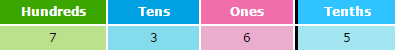In 736.5,
• the digit 7 is in the hundreds place, its value is 7 × 100 or 700
• the digit 3 is in the tens place, its value is 3 × 10 or 30
• the digit 6 is in the ones place, its value is 6 × 1 or 6
• the digit 5 is in the tenths place, its value is 5 × 0.1 or 0.5
700   +   30   +   6   +   0.5  =  736.5

#### 5. Understanding Hundredths

Divide 1 whole into 100 equal parts.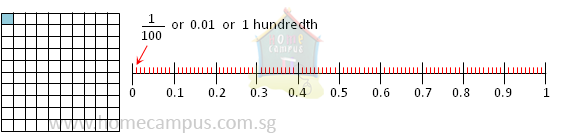In the fraction form, each part has a value equal to
 1 100
or 1 hundredth.

In the decimal form, each part has a value equal to 0.01 (read as zero point zero one).

#### 6. What decimals do the following shaded parts represent?

a)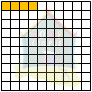4 hundredths
 4 100
=   0.04
b)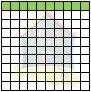10 hundredths
10 hundredths  =  1 tenth
=  0.1
10 hundredths make 1 tenth.
c)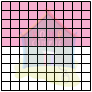50 hundredths
 50 100
=   0.50

Or,

 50 100
=
 5 10

=    0.5

The decimals 0.5 and 0.50 have the same value.
d)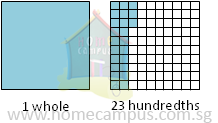1 23 100
=   1  +
 23 100

=  1  +  0.23
=  1.23
e)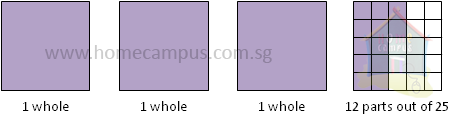3 12 25
=   3  +
 12 25

=   3  +
 48 100

=   3  +  0.48
=   3.48
Change the denominator to a multiple of 10.

25  ×  4 = 100

#### 7. What fractions or mixed numbers do the following decimals represent? Express in the simplest form.

a)   0.03
0.03  =
 3 100
b)   0.5
0.25  =
 25 100

 1 4
c)   1.55
1.55  =
 1 55 100

=
 1 11 20

#### 8. What is the value of each digit in 73.65?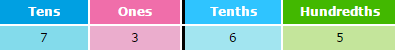In 73.65,
• the digit 7 is in the tens place, its value is 7 × 10 or 70
• the digit 3 is in the ones place, its value is 3 × 1 or 3
• the digit 6 is in the tenths place, its value is 6 × 0.1 or 0.6
• the digit 5 is in the hundredths place, its value is 5 × 0.01 or 0.05
70   +   3   +   0.6   +   0.05  =  73.65

#### 9. What is the value of each digit in 73.65?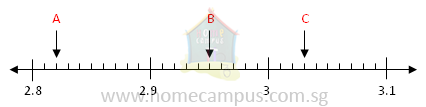A  =  2.82
B  =  2.95
C  =  3.03

#### 10. Do the following.

a)   Change \$10 and 35 ¢ to dollars.

\$10   and   35 ¢  =  \$10  +  \$0.35
=  \$10.35
100 ¢  =  \$1
1 ¢  =
 1 100
of \$1

=  \$0.01

b)   Write 4 m 65 cm in m.

4 m   65 cm  =  4 m  +  65 cm
=  4.65 m
100 cm  =  1 m
1 cm  =
 1 100
m

=  0.01 m
c)   Express
 9 3 100
litres in decimal.

 9 3 100
L   =   9 L  +
 3 100
L
=   9 L   +   0.03 L
=   9.03 L

#### 11. Understanding Thousandths

Divide 1 whole into 1000 equal parts.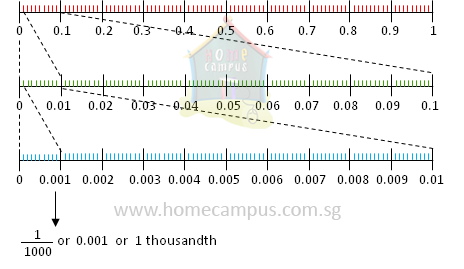In the fraction form, each part has a value equal to
 1 1000
or 1 thousandth.

In the decimal form, each part has a value equal to 0.001 (read as zero point zero zero one).

#### 12. What decimals do the following fractions or mixed numbers represent?

a)   8 thousandths
8 thousandths  =
 8 1000
=  0.008
b)
 2607 1000

 2607 1000
=
 2000 1000
+
 607 1000

=  2  +  0.607
=  2.607
c)
 5 300 1000

 5 300 1000
=   5  +
 300 1000

=   5  +  0.300
=   5.300

Or,

 5 300 1000
=   5  +
 300 1000

=   5  +
 3 10

=   5  +  0.3
=   5.3

The decimals 5.300 and 5.3 have the same value.

#### 13. What fractions or mixed numbers do the following decimals represent? Express in the simplest form.

a)   0.003
0.003   =
 3 1000
b)   0.250
0.250   =
 250 1000

=
 25 100

=
 1 4
c)   1.775
1.775   =
 1 775 1000

=
 1 33 40

#### 14. What is the value of each digit in 7.365?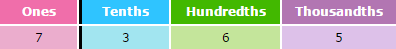In 7.365,
• the digit 7 is in the ones place, its value is 7 × 1 or 7
• the digit 3 is in the tenths place, its value is 3 × 0.1 or 0.3
• the digit 6 is in the hundredths place, its value is 6 × 0.01 or 0.06
• the digit 5 is in the thousandths place, its value is 5 × 0.001 or 0.005
7   +   0.3   +   0.06   +   0.005   =   7.365

#### 15. Write a decimal for each letter on the number line below.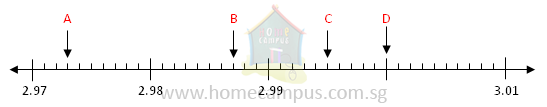A  =  2.973
B  =  2.988
C  =  2.995
D  =  3

#### 1 Whole

1  =
 10 10
=
 100 100
=
 1000 1000

Or,

1 whole  =  10 tenths
1 whole  =  100 hundredths
1 whole  =  1000 thousandths

1 tenth  =  0.1
1 hundredth  =  0.01
1 thousandth  =  0.001

#### 16. Compare the numbers 3.687, 3.678 and 3.876 and 3.786. a) Which number is the smallest? b) Which number is the greatest? c) Arrange the numbers in increasing order. d) Arrange the numbers in decreasing order.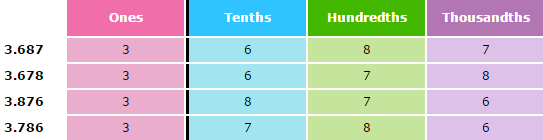a)   3.678 is the smallest number.

b)   3.876 is the greatest number.

c)   Increasing order:
3.678,    3.687,    3.786,    3.876

d)   Decreasing order:
3.876,    3.786,    3.687,    3.678
Compare the numbers digit by digit starting with the digit in the highest place value.

#### 17. Complete the number patterns below. a)    3.591,    3.594,    ______,    3.6,    ______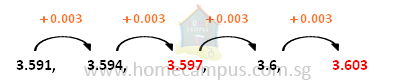Rule: Add 0.003 to get the next decimal number.

#### b)    14.72,    13.62,    ______,    11.42,    ______,    9.22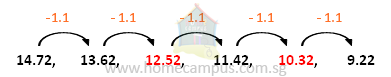Rule: Subtract 1.1 to get the next decimal.